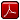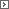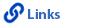Mathematics Grade 3Printable Version (pdf)Course Introduction

Core Standards of the Course

Strand: MATHEMATICAL PRACTICES (3.MP)
The Standards for Mathematical Practice in Third Grade describe mathematical habits of mind that teachers should seek to develop in their students. Students become mathematically proficient in engaging with mathematical content and concepts as they learn, experience, and apply these skills and attitudes (Standards 3.MP.1�8).Standard 3.MP.1
Make sense of problems and persevere in solving them. Explain the meaning of a problem, look for entry points to begin work on the problem, and plan and choose a solution pathway. When a solution pathway does not make sense, look for another pathway that does. Explain connections between various solution strategies and representations. Upon finding a solution, look back at the problem to determine whether the solution is reasonable and accurate, often checking answers to problems using a different method or approach.

Standard 3.MP.2
Reason abstractly and quantitatively. Make sense of quantities and their relationships in problem situations. Contextualize quantities and operations by using images or stories. Decontextualize a given situation and represent it symbolically. Interpret symbols as having meaning, not just as directions to carry out a procedure. Know and flexibly use different properties of operations, numbers, and geometric objects.

Standard 3.MP.3
Construct viable arguments and critique the reasoning of others. Use stated assumptions, definitions, and previously established results to construct arguments. Explain and justify the mathematical reasoning underlying a strategy, solution, or conjecture by using concrete referents such as objects, drawings, diagrams, and actions. Listen to or read the arguments of others, decide whether they make sense, ask useful questions to clarify or improve the arguments, and build on those arguments.

Standard 3.MP.4
Model with mathematics. Identify the mathematical elements of a situation and create a mathematical model that shows the relationships among them. Identify important quantities in a contextual situation, use mathematical models to show the relationships of those quantities, analyze the relationships, and draw conclusions. Models may be verbal, contextual, visual, symbolic, or physical.

Standard 3.MP.5
Use appropriate tools strategically. Consider the tools that are available when solving a mathematical problem, whether in a real-world or mathematical context. Choose tools that are relevant and useful to the problem at hand, such as drawings, diagrams, technologies, and physical objects and tools, as well as mathematical tools such as estimation or a particular strategy or algorithm.

Standard 3.MP.6
Attend to precision. Communicate precisely to others by crafting careful explanations that communicate mathematical reasoning by referring specifically to each important mathematical element, describing the relationships among them, and connecting their words clearly to representations. Calculate accurately and efficiently, and use clear and concise notation to record work.

Standard 3.MP.7
Look for and make use of structure. Recognize and apply the structures of mathematics such as patterns, place value, the properties of operations, or the flexibility of numbers. See complicated things as single objects or as being composed of several objects.

Standard 3.MP.8
Look for and express regularity in repeated reasoning. Notice repetitions in mathematics when solving multiple related problems. Use observations and reasoning to find shortcuts or generalizations. Evaluate the reasonableness of intermediate results.

Strand: OPERATIONS AND ALGEBRAIC THINKING (3.OA)
Represent and solve problems involving multiplication and division within 100 (Standards 3.OA.1�4 and Standard 3.OA.7). They demonstrate understanding of the properties of multiplication and the relationship between multiplication and division (Standards 3.OA.5�6). Students use the four operations to identify and explain patterns in arithmetic (Standards 3.OA.8�9).Standard 3.OA.1
Interpret products of whole numbers, such as interpret 5 � 7 as the total number of objects in 5 groups of 7 objects each. For example, describe a context in which a total number of objects can be expressed as 5 � 7.Standard 3.OA.2
Interpret whole-number quotients of whole numbers. For example, interpret 56 � 8 as the number of objects in each share when 56 objects are partitioned equally into eight shares (partitive), or as a number of shares when 56 objects are partitioned into equal shares of eight objects each (quotative).Standard 3.OA.3
Use multiplication and division within 100 to solve word problems in situations involving equal groups, arrays, and measurement quantities. For example, use drawings and equations with a symbol for the unknown number to represent the problem.Standard 3.OA.4
Determine the unknown whole number in a multiplication or division equation relating three whole numbers. For example, determine the unknown number - product, factor, quotient, dividend, or divisor - that makes the equation true in each of the equations 8 x ? = 48, 5 = ? � 3, 6 x 6 = ?.Standard 3.OA.5
Apply properties of operations as strategies to multiply and divide. For example: If 6 x 4 = 24 is known, then 4 x 6 = 24 is also known (commutative property of multiplication). 3 x 5 x 2 can be found by 3 x 5 = 15, then 15 x 2 = 30, or by 5 x 2 = 10, then 3 x 10 = 30 (associative property of multiplication). Knowing that 8 x 5 = 40 and 8 x 2 = 16, one can find 8 x 7 as 8 x (5 + 2) = (8 x 5) + (8 x 2) = 40 + 16 = 56 (distributive property). (Third grade students may, but need not, use formal terms for these properties.)Standard 3.OA.6
Understand division as an unknown-factor problem. Understand the relationship between multiplication and division (multiplication and division are inverse operations). For example, find 32 � 8 by finding the number that makes 32 when multiplied by 8.Standard 3.OA.7
Fluently multiply and divide.1. Fluently multiply and divide within 100, using strategies such as the relationship between multiplication and division or properties of operations. (For example, knowing that 8 x 5 = 40, one knows 40 � 5 = 8).

Standard 3.OA.8
Solve two-step word problems.1. Solve two-step word problems using the four operations. Know how to perform operations in the conventional order when there are no parentheses to specify a particular order (Order of Operations). (Limit to problems posed with whole numbers and having whole number answers.)
2. Represent two-step problems using equations with a letter standing for the unknown quantity. Create accurate equations to match word problems.
3. Assess the reasonableness of answers using mental computation and estimation strategies, including rounding.

Standard 3.OA.9
Identify arithmetic patterns (including patterns in the addition table or multiplication table), and explain them using properties of operations. For example, observe that four times a number is always even, and explain why four times a number can be decomposed into two equal addends.Strand: NUMBER AND OPERATIONS IN BASE TEN (3.NBT)
Use place value understanding and properties of operations to perform multi-digit arithmetic. A range of algorithms may be used (Standards 3.NBT.1�3).Standard 3.NBT.1
Use place value understanding to round whole numbers to the nearest 10 or 100.Standard 3.NBT.2
Fluently add and subtract within 1,000 using strategies and algorithms based on place value, properties of operations, and/or the relationship between addition and subtraction.Standard 3.NBT.3
Multiply one-digit whole numbers by multiples of 10 in the range 10�90 (for example, 9 x 80 and 5 x 60) using strategies based on place value and properties of operations.Strand: NUMBER AND OPERATIONS - FRACTIONS (3.NF)
Develop understanding of fractions as numbers. Denominators are limited to 2, 3, 4, 6, and 8 in third grade.Standard 3.NF.1
Understand that a unit fraction has a numerator of one and a non-zero denominator.1. Understand a fraction 1/b as the quantity formed by one part when a whole is partitioned into b equal parts.
2. Understand a fraction a/b as the quantity formed by a parts of size 1/b. For example: 1/4 + 1/4 + 1/4 = 3/4.

Standard 3.NF.2
Understand a fraction as a number on the number line; represent fractions on a number line diagram.1. Represent a fraction 1/b on a number line diagram by defining the interval from 0 to 1 as the whole and partitioning it into b equal parts. Recognize that each part has size 1/b and that the endpoint of the part based at 0 locates the number 1/b on the number line.
2. Represent a fraction a/b on a number line diagram by marking off a lengths 1/b from 0. Recognize that the resulting interval has size a/b and that its endpoint locates the number a/b on the number line.

Standard 3.NF.3
Explain equivalence of fractions in special cases, and compare fractions by reasoning about their size.1. Understand two fractions as equivalent if they are the same size, or the same point on a number line.
2. Recognize and generate simple equivalent fractions, such as 1/2 = 2/4, 4/6 = 2/3. Explain why the fractions are equivalent by using a visual fraction model, for example.
3. Express whole numbers as fractions, and recognize fractions that are equivalent to whole numbers. For example, express 3 in the form 3 = 3/1; recognize that 6/1 = 6; locate 4/4 and 1 at the same point of a number line diagram.
4. Compare two fractions with the same numerator or the same denominator by reasoning about their size. Recognize that comparisons are valid only when the two fractions refer to the same whole. Record the results of comparisons with the symbols >, =, or <, and justify the conclusions, for example, by using a visual fraction model.

Strand: MEASUREMENT AND DATA (3.MD)
Solve problems involving measurement and estimation of intervals of time, liquid volumes, and masses of objects. (Standards 1�2). Represent and interpret data (Standards 3.MD.3� 4). Understand concepts of area and relate area to multiplication and addition (Standards 3.MD.5�7). Recognize perimeter as an attribute of plane figures and distinguish between linear and area measures (Standard 3.MD.8).Standard 3.MD.1
Tell and write time to the nearest minute and measure time intervals in minutes. Solve word problems involving addition and subtraction of time intervals in minutes, for example, by representing the problem on a number line diagram.Standard 3.MD.2
Measure and estimate liquid volumes and masses of objects using standard units of grams (g), kilograms (kg), milliliters (ml), and liters (l). (Excludes compound units such as cubic centimeters [cc or cm3] and finding the geometric volume of a container.) Add, subtract, multiply, or divide to solve one-step word problems involving masses of objects or volumes of liquids that are given in the same units, for example, by using drawings (such as a beaker with a measurement scale) to represent the problem. (Excludes multiplicative comparison problems.)Standard 3.MD.3
Draw a scaled picture graph and a scaled bar graph to represent a data set with several categories. Solve one- and two-step "how many more" and "how many less" problems using information presented in scaled bar graphs. For example, draw a bar graph in which each square in the bar graph might represent five pets.Standard 3.MD.4
Generate measurement data by measuring lengths using rulers marked with halves and fourths of an inch. Show the data by making a line plot where the horizontal scale is marked off in appropriate units-whole numbers, halves, or quarters.Standard 3.MD.5
Recognize area as an attribute of plane figures and understand concepts of area measurement.1. A square with side length one unit, called "a unit square," is said to have "one square unit" of area, and can be used to measure area.
2. A plane figure which can be covered without gaps or overlaps by n unit squares is said to have an area of n square units.

Standard 3.MD.6
Measure area by counting unit squares (square centimeters, square meters, square inches, square feet, and improvised units).Standard 3.MD.7
Relate area to the operations of multiplication and addition (refer to 3.OA.5).1. Find the area of a rectangle with whole-number side lengths by tiling it, and show that the area is the same as would be found by multiplying the side lengths.
2. Multiply side lengths to find areas of rectangles with whole-number side lengths in the context of solving real-world and mathematical problems, and represent wholenumber products as rectangular areas in mathematical reasoning.
3. Use tiling to show in a concrete case that the area of a rectangle with whole-number side lengths a and b + c is the sum of a x b and a x c. Use area models to represent the distributive property in mathematical reasoning.
4. Recognize area as additive. Find areas of rectilinear figures by decomposing them into non- overlapping rectangles and adding the areas of the non-overlapping parts, applying this technique to solve real-world problems.

Standard 3.MD.8
Solve real-world and mathematical problems involving perimeters of polygons, including finding the perimeter given the side lengths, finding an unknown side length, and exhibiting rectangles with the same perimeter and different areas or with the same area and different perimeters.Strand: GEOMETRY (3.G)
Reason with shapes and their attributes (Standards 3.G.1�2).Standard 3.G.1
Understand that shapes in different categories (for example, rhombuses, rectangles, and others) may share attributes (for example, having four sides), and that the shared attributes can define a larger category (for example, quadrilaterals). Recognize rhombuses, rectangles, and squares as examples of quadrilaterals, and draw examples of quadrilaterals that do not belong to any of these subcategories.Standard 3.G.2
Partition shapes into parts with equal areas. Express the area of each part as a unit fraction of the whole. For example, partition a shape into four parts with equal area, and describe the area of each part as 1/4 of the area of the shape.http://www.uen.org - in partnership with Utah State Board of Education (USBE) and Utah System of Higher Education (USHE).  Send questions or comments to USBE Specialist - Shannon Olson and see the Mathematics - Elementary website. For general questions about Utah's Core Standards contact the Director - Jennifer Throndsen .

These materials have been produced by and for the teachers of the State of Utah. Copies of these materials may be freely reproduced for teacher and classroom use. When distributing these materials, credit should be given to Utah State Board of Education. These materials may not be published, in whole or part, or in any other format, without the written permission of the Utah State Board of Education, 250 East 500 South, PO Box 144200, Salt Lake City, Utah 84114-4200.